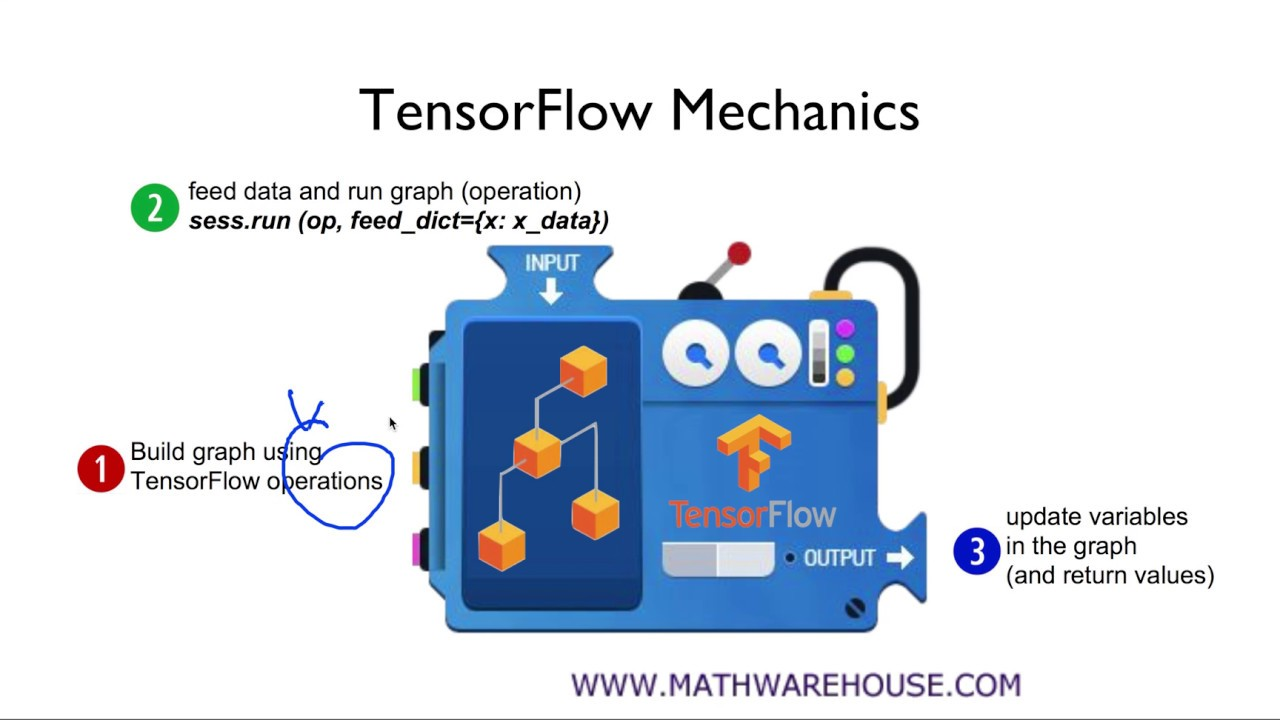CLOSE

Recommanded Free YOUTUBE Lecture: <% selectedImage %>

## Contents

### Lenear Regression

이전 장 ML의 기본 컨셉에서 머신러닝이 무엇인지와 머신러닝에서 사용하는 기본적인 용어들에 대해서 살펴봤다. 이번 장에서는 머신러닝에서 널리 사용하는 선형회귀(lenear regression)를 살펴볼 것이다.

공부시간에 따른 예상 성적을 알려주는 인공지능 프로그램을 만든다고 가정해보자.머신러닝을 위한 "훈련데이터"를 준비했다. X축은 공부시간이고, Y축은 예상성적이다. 이 데이터들을 학습시키면, 훈련 데이터를 가장 잘 표현 할 수 있는 어떤 "모델"이 만들어진다. 모델은 어떤 값을 집어 넣으면, 그 결과가 튀어나오는 "함수"라고 생각 할 수 있다. 모델이 만들어지면, 여기에 X 값을 넣는 것만으로 결과를 예측 할 수 있다. 7시간 공부한 학생이라면 대략 65 점 정도가 나올 거라고 예상 할 수 있다.

훈련데이터가 주어지므로 슈퍼바이저 타입이며, 값이 0 ~ 100까지의 범위를 가지므로 regression 타입의 머신러닝 문제다.이런 단순한 데이터는, 직선을 하나 그어주는 것만으로도 눈짐작으로 대략 모델을 만들 수 있을 것이다. 하지만 데이터가 복잡해지면 눈짐작으로는 한계가 있을 것이다. 우리는 눈 짐작이 아닌, 여러 상황에 자유롭게 적용 가능한 모델을 만들 수 있는 방법을 찾아야 한다.

설명을 간단하게 하기 위해서 훈련데이터를 더욱 간단하게 했다.
 x y 1 1 2 2 3 3
x가 1일때 y가 1, 2->2, 3->3인 간단한 데이터가 있다고 가정해보자. 이것을 그래프로 나타내 보자.Regression 모델을 학습하기 위해서는 하나의 가설(Hypothesis)를 세워야 한다. 학습할 데이터를 보아하니, 이렇게 하면 원하는 모델을 만들 수 있겠군!! 하는 그 지점을 찾아내는 거다. 여기에서 학습데이터를 보면 Lenear 한 가설을 세우면 모델을 만들 수 있을 것 같다는 느낌이 들 것이다. 즉 아래와 같이 여러 개의 선을 그려서 가장 적당한 가설을 찾는 것이다.이걸 2차원에서 수학적으로 가장 잘 나타낼려먼 어떻게 해야 할까 ? 많이 봤던 형태의 일차방정식으로 표현할 수 있을 것이다. 이제 우리는 W와 b를 조절해서, 데이터를 가장 잘 모델링하는 가설을 만들 수 있을 것이다. 위 그래프에는 총 3개의 가설이 있다.

### Cost function

위의 3개의 가설 중 가장 좋은 가설인지 어떻게 알 수 있을가 ? "가설"과 훈련 데이터의 거리를 비교해서 거리의 차가 가장 작다면, 가장 좋은 모델이라고 말 할 수 있을 것이다.이 거리를 계산하는 함수를 Cost function 혹은 Loss function 이라고 한다. 차이를 계산하는 가장 간단한 함수는 다. 간단하긴 하지만 좋은 모델은 아니다. 값이 양수일수도 있고, 음수일 수도 있기 때문이다. 보통은 거리에 제곱을 한다. 제곱을 해주면 모든 값이 양수가 되며, 작은 차이를 크게 만들어주기 때문에 연산하기가 쉬워진다. 이 함수가 cost function 이다. 공식을 모든 훈련 데이터에 대해서 일반화 해보자.

모든 훈련 데이터에 대한 거리를 제곱해서 더하고, 훈련데이터의 갯수만큼으로 나눴다. 데이터가 3개인 경우에는 위와 같이 표현 할 수도 있겠으나, 데이터가 수천, 수만이라면 표현이 힘들 것이다. 그래서 좀더 일반화 했다.

이렇게 우리는 cost function

과 가설 을 얻었다. 여기에서 cost function은 W와 b에 대한 함수라는 걸 알 수 있다.

Linear regression의 숙제는 가장 작은 값을 가지는 W와 b를 구하는 것이다. 여기에서 아래와 같은 학습목표를 세울 수 있다. cost의 값을 최소화 하는 다양한 알고리즘이 있는데, 다음 장에서 살펴보도록 하겠다.

### TensorFlow를 이용한 구현

#### Hypothesis and cost function

선형회귀(Linear regression)문제를 풀기 위한 hypothesis 와 cost function을 아래와 같이 정의했다. 우리가 새운 가설을 만족하도록 가장 작은 cost function을 만드는 과정이 학습이다. TensorFlow를 이용해서 문제를 풀어보자. 텐서플로우는 3개의 스텝으로 작동한다.1. 텐서플로우는 먼저 그래프를 빌드한다.
2. 그래프 빌드가 끝나면 sess.run으로 그래프를 실행한다.
3. 실행이 끝나면, 결과가 나오거나 그래프의 variables를 업데이트 한다.

#### TF를 이용해서 그래프를 만들기

가설과 cost function을 텐서플로우를 이용해서 코드로 표현하면 된다. 먼저 가설 의 텐서플로우 코드다.
# x and y data
x_train = [1,2,3]
y_train = [1,2,3]

W= tf.Variable(tf.random_normal(), name='weight')
b= tf.Variable(tf.random_normal(), name='bias')

hypothesis = x_train * W + b
...계속